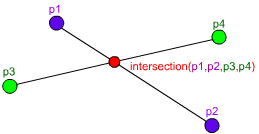## Line-Line Intersection in C++I’ve just been tasked with creating a function to get the intersection of two lines.
With the help of equations from Wolfram Mathworld I created this nifty function:

```Point* intersection(Point p1, Point p2, Point p3, Point p4) {
// Store the values for fast access and easy
// equations-to-code conversion
float x1 = p1.x, x2 = p2.x, x3 = p3.x, x4 = p4.x;
float y1 = p1.y, y2 = p2.y, y3 = p3.y, y4 = p4.y;

float d = (x1 - x2) * (y3 - y4) - (y1 - y2) * (x3 - x4);
// If d is zero, there is no intersection
if (d == 0) return NULL;

// Get the x and y
float pre = (x1*y2 - y1*x2), post = (x3*y4 - y3*x4);
float x = ( pre * (x3 - x4) - (x1 - x2) * post ) / d;
float y = ( pre * (y3 - y4) - (y1 - y2) * post ) / d;

// Check if the x and y coordinates are within both lines
if ( x < min(x1, x2) || x > max(x1, x2) ||
x < min(x3, x4) || x > max(x3, x4) ) return NULL;
if ( y < min(y1, y2) || y > max(y1, y2) ||
y < min(y3, y4) || y > max(y3, y4) ) return NULL;

// Return the point of intersection
Point* ret = new Point();
ret->x = x;
ret->y = y;
return ret;
}```

Hope it will be to as good use to you as it is to me.

### 9 Responses to “Line-Line Intersection in C++”

1.Phloxi Says:

You bloody beauty.

2.BNelsey Says:

still learning C++ and this definitely helped me, thanks a bunch!

3.Brian Washechek Says:

Here is mycode
bool intersection(float x1,float y1,
float x2,float y2,
float x3,float y3,
float x4,float y4) {
// Store the values for fast access and easy

// equations-to-code conversion

//float x1 = p1.x, x2 = p2.x, x3 = p3.x, x4 = p4.x;
//float y1 = p1.y, y2 = p2.y, y3 = p3.y, y4 = p4.y;

float d = (x1 – x2) * (y3 – y4) – (y1 – y2) * (x3 – x4);
// If d is zero, there is no intersection

if (d == 0) return false;

// Get the x and y

float pre = (x1*y2 – y1*x2), post = (x3*y4 – y3*x4);

float x = ( pre * (x3 – x4) – (x1 – x2) * post ) / d;
float y = ( pre * (y3 – y4) – (y1 – y2) * post ) / d;

// Check if the x and y coordinates are within both lines

if ( x max(x1, x2) ||
x max(x3, x4) ) return false;
if ( y max(y1, y2) ||
y max(y3, y4) ) return false;

// Return the point of intersection
//But I don’t care about “where”, only “if”
//Point* ret = new Point();
//ret->x = x;
//ret->y = y;

return true;
}

void fixCollisions()
{
bigX=true; //Here’s the problem. Not with the hit detection math, but with the display
//of results
for (int i =0;i<wallUbound;i++)
{

if (intersection(FDX-cos(FDA),FDY-sin(FDA), //<–Right here
FDX+cos(FDA)+10,FDY+sin(FDA),
wallX[i]-cos(wallRot[i]),wallY[i]-sin(wallRot[i]),
wallX[i]+cos(wallRot[i]),wallY[i]+sin(wallRot[i])))
bigX=true;

}

}

4.Stephen Says:

Your test for x & y being within the lines extent will often fail when one or other of the lines is either horizontal or vertical due to floating point rounding errors.

A better test would be:
if (x (std::max(x1, x2) + epsilon) ||
x (std::max(x3, x4) + epsilon))
return false;
if (y (std::max(y1, y2) + epsilon) ||
y (std::max(y3, y4) + epsilon))
return false;

Where epsilon is say, 1e-5. This has the effect of testing against a line with a thickness of 2*epsilon accommodating any rounding errors.

5.Stephen Says:

Lets try that again taking account of HTML :)

if (x < (min(x1, x2) – epsilon) ||
x > (max(x1, x2) + epsilon) ||
x < (min(x3, x4) – epsilon) ||
x > (max(x3, x4) + epsilon))
return false;
if (y < (min(y1, y2) – epsilon) ||
y > (max(y1, y2) + epsilon) ||
y < (min(y3, y4) – epsilon) ||
y > (max(y3, y4) + epsilon))
return false;

6.varsha Says:

@Brian Washechek
I ahve problen in C
I am trying to find intersection of multilines but failed it only gives 1st two

how should i check for multiple line intersection if I return boolean status i can check but how to reshuffle the end point each time

7.Brian Washechek Says:

I don’t get it. Are you making fun of how little I know?

8.David Sopala Says:

Don’t return a bool to check multiple line intersections, you MUST return a point. When you have a point from the first two lines check to see if the point is valid for the 3,4,5…n lines if all return true then yes they all intersect.

9.Niko Says:

Thanks! AND Thanks Stephen!

I was writing a path planning algorithm where the obstacles were all up-right rectangles so at least one of the two lines I passed to this function would always be either horizontal or vertical. It would have taken me way too long to figure out what was wrong if I didn’t see your post.